﻿ 一种TI介质纯qP波正演方法及其在逆时偏移中的应用
 地球物理学报2017, Vol. 60Issue (11): 4447-4467PDF

Pure quasi-P wave forward modeling method in TI media and its application to RTM
YANG Peng, LI Zhen-Chun, GU Bing-Luo
School of Geosciences, China University of Petroleum (East China), Shandong Qingdao 266580, China
Abstract: Based on the exact dispersion relation proposed by Tsvankin, and adopting the approximate expanded method, this work derives the decoupled pure quasi-P wave equations in a TTI medium. Then we divide the pseudodifferential operator in the equations into a Laplace operator and a scalar operator to indicate the exact transmission direction of qP waves, and establish second-order pure qP wave equations in the time domain. In this induction process, there is no need to set S wave velocity at 0, which can describe the kinetic features of qP waves in a more accurate way. Compared with the second-order decoupled qP wave equations in the wavenumber domain, this method has a higher computation efficiency and lower storage demand. While compared with the second-order pure qP wave equations in the time domain which is based on derivation from Alkhalifah dispersion relation, it has better suppression of artifacts and less numeral error, being more general. But using this method to solve wave vectors is asymptotic approximation to adopting first-order of wavefield gradients, which might cause inaccurate amplitude of qP waves perpendicular to the axis. In order to correct amplitude and apply the elliptical decomposition method to these equations, pure qP wave elliptical decomposition equations have been derived, achieving more balanced amplitude. Compared with equations proposed by Xu, the wavefield amplitude derived from pure qP wave elliptical decomposition equations established in this paper is more accurate. Firstly, we choose a homogeneous TTI medium to conduct forward modeling of qP waves, and analyze the amplitude of single trace of the qP wavefield, verifying the correctness and effectiveness of the qP wave equations and qP wave elliptical decomposition equations constructed in this paper. Then, we choose the BP TTI model to conduct forward modeling of qP waves, and combine the results of stimulation and amplitude analysis of a homogeneous TTI medium, highlighting the advantages and adaptability of qP wave elliptical decomposition equations established in this paper. Lastly, we apply the qP wave elliptical decomposition wave equations derived in this paper to implement reverse time migration imaging for a thrust model and BP TTI model, which verifies feasibility of the equations and its applicability in RTM.
Key words: TI media    Pure qP waves    Dispersion relation    Elliptical decomposition    Reverse time migration
1 引言

2 各向异性介质纯qP波方程

Alkhalifah(2000)提出了声学近似频散关系，将沿着对称轴方向的横波速度设置为零，构建声学近似方程，忽略横波物理现象，描述qP波在各向异性介质中的传播特征.Alkhalifah提出的声学近似频散关系为：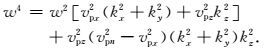(1)

Liu等(2009)通过开平方根求解得到纵、横波频散关系式，分别为：

qP波：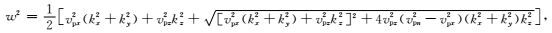(2)

qSV波：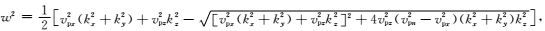(3)

qP波：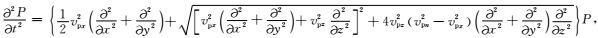(4)

qSV波：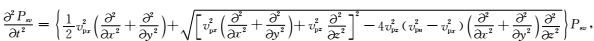(5)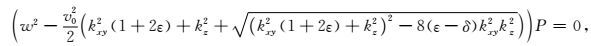(6)(7)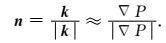(8)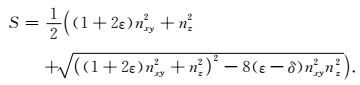(9)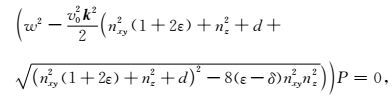(10)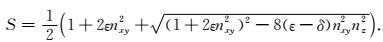(11)

Xu等(2014)利用方程(11)求得的标量算子构建了时间域二阶纯qP波方程，可以很好地压制干扰，减小数值误差，保证数值计算稳定，较精确地描述了qP波的传播特征，但是只有加入衰减项d，才能很好地压制干扰(见图 3).由于衰减项的引入，在构建纯qP波椭圆分解方程(Xu et al., 2015)进行振幅校正时，进而造成振幅不准确，振幅值偏小，下文详细说明(见图 89).因此为了避免引入衰减项，本文将Xu和Zhou(2014)采用的Alkhalifah(2000)提出的声学近似频散关系换为Tsvankin(1996)提出的精确频散关系，按照上述Xu和Zhou(2014)求取标量算子S的方法来求取本文所采用的标量算子S，然后构建时间域二阶纯qP波方程，无需引入衰减项，数值计算稳定，误差小，振幅更加准确.首先需求精确频散关系，Tsvankin(1996)采用的P波和SV波的相速度表达式为：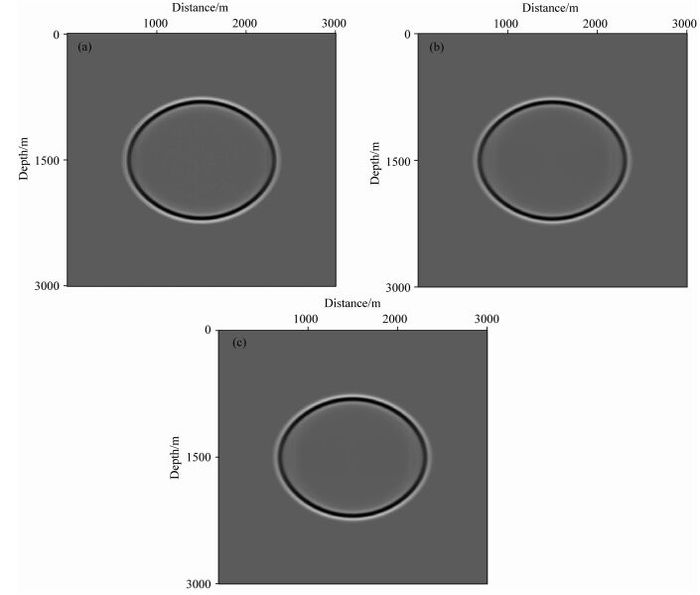图 3 沿y轴中心方向qP波波场快照的二维切片 (a) qP波原始方程(方程(9)和(25))；(b) Xu和Zhou(2014)的方程(方程(11)和(25))；(c)本文方程(方程(23)和(25)). Fig. 3 2D seismic slices of snapshot of qP wavefield along the middle of y direction (a) Original qP wave equation(Equations (9) and (25)); (b) Xu′s equation (Equations (11) and (25)); (c) Equations proposed in this paper (Equations (23) and (25)).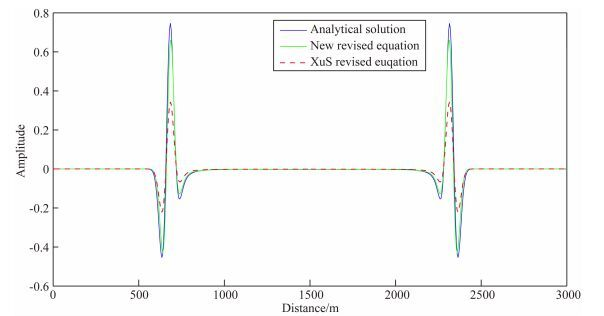图 8 z=1.5 km处沿水平方向qP波场的单道波形图 蓝线：解析解方程；红虚线：Xu等(2015)的椭圆分解方程；绿线：本文椭圆分解方程. Fig. 8 Waveforms of qP wavefield at z=1.5 km in horizontal direction Blue solid line: analytical equation; Red dash line: Xu′s elliptical decomposed equation; Green solid line: elliptical decomposed equation proposed in this paper.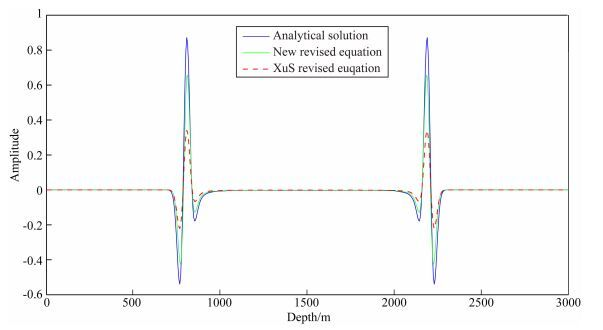图 9 x=1.5 km处沿垂直方向qP波场的单道波形图 蓝线：解析解方程；红虚线：Xu等(2015)的椭圆分解方程；绿线：本文椭圆分解方程. Fig. 9 Waveforms of qP wavefield at x=1.5 km in depth direction Blue solid line: analytical equation; Red dash line: Xu′s elliptical decomposed equation; Green solid line: elliptical decomposed equation proposed in this paper.

P波：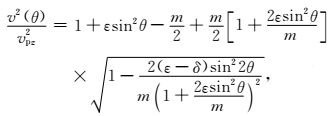(12)

SV波：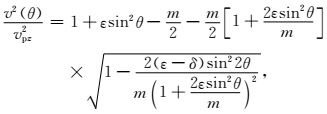(13)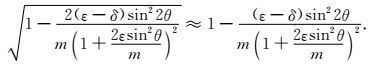(14)

P波：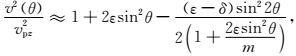(15)

SV波：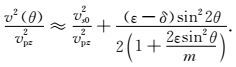(16)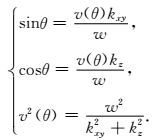(17)

P波：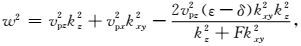(18)

SV波：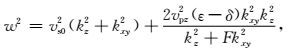(19)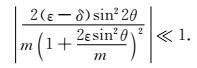(20)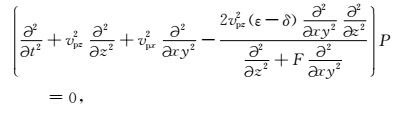(21)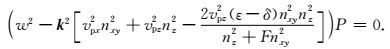(22)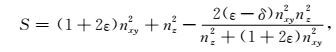(23)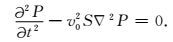(24)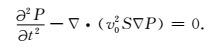(25)

3 纯qP波椭圆分解方程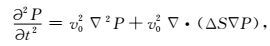(26)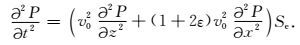(27)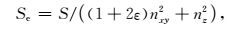(28)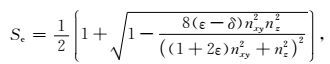(29)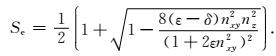(30)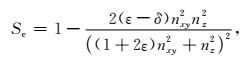(31)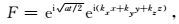(32)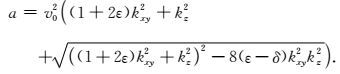(33)

4 模型试算

4.1 均匀各向异性介质模型正演模拟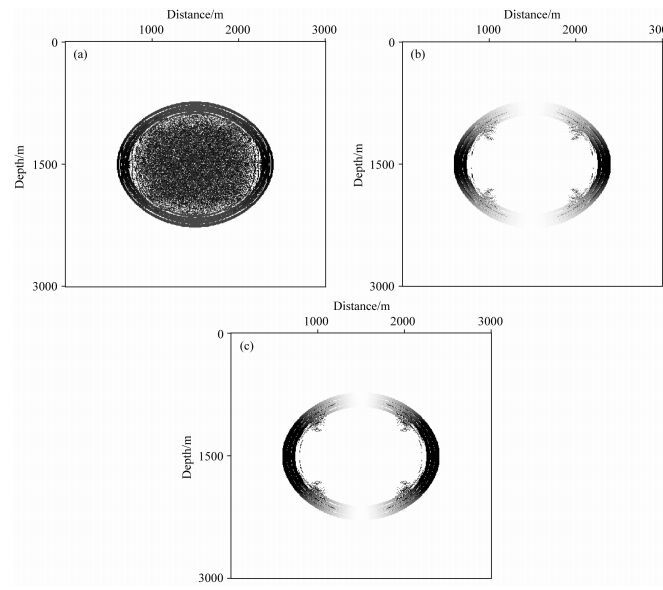图 1 沿y轴中心方向标量算子S的二维切片 (a) qP波原始方程(方程(9)和(25))；(b) Xu和Zhou(2014)的方程(方程(11)和(25))；(c)本文方程(方程(23)和(25)). Fig. 1 2D seismic slices of scalar operator S along the middle of y direction (a) qP wave original equation (Equations (9) and (25)); (b) Xu′s equation (Equations (11) and (25)); (c) Equations proposed in this paper (Equations (23) and (25)).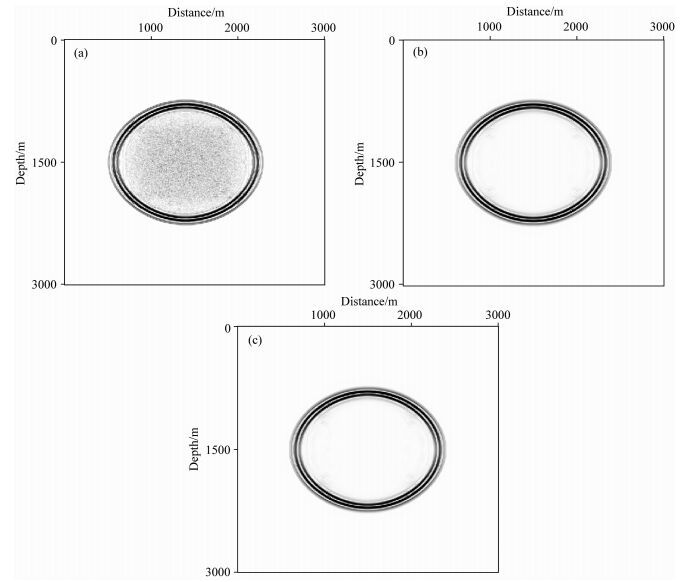图 2 沿y轴中心方向复合算子S∇P的二维切片 (a) qP波原始方程(方程(9)和(25))；(b) Xu等(2014)的方程(方程(11)和(25))；(c)本文方程(方程(23)和(25)). Fig. 2 2D seismic slices of composition operator S∇P along the middle of y direction (a) Original qP wave equation (Equations (9) and (25)); (b) Xu′s equation (Equations (11) and (25)); (c) Equations proposed in this paper (Equations (23) and (25)).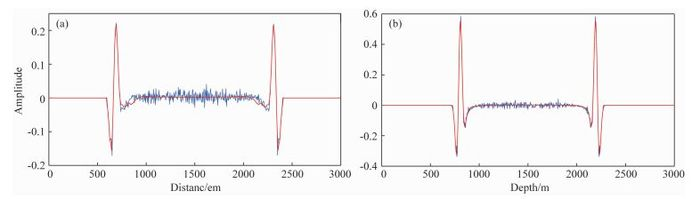图 4 qP波场的单道波形对比1 (a) z=1.5 km处沿x方向抽取的单道波形；(b) x=1.5 km处沿z向抽取的单道波形.红虚线：Xu和Zhou(2014)方程(方程(11)和(25))蓝线：qP波原始方程(方程(9)和(25)). Fig. 4 First comparison of single trace of qP wavefield (a) Waveforms of single trace of qP wavefield at z=1.5 km in x direction; (b) Waveforms of single trace of qP wavefield at x=1.5 km in z direction. Red dash line: Xu′s equation(Equations (11) and (25)); blue solid line: original qP wave equation (Equations (9) and (25)).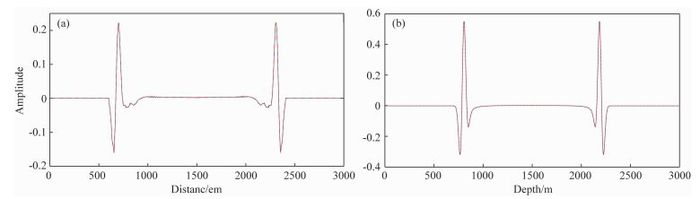图 5 qP波场单道波形对比2 (a) z=1.5 km处沿x方向抽取的单道波形；(b) x=1.5 km处沿z向抽取的单道波形.红虚线：Xu和Zhou(2014)方程(方程(11)和(25))蓝线：本文方程(方程(23)和(25)). Fig. 5 Second comparison of single trace of qP wavefield (a) Waveforms of single trace of qP wavefield at z=1.5 km in x direction; (b) Waveforms of single trace of qP wavefield at x=1.5 km in z direction. Red dash line: Xu′s equation (Equations (11) and (25)); blue solid line: equations proposed in this paper(Equations (23) and (25)).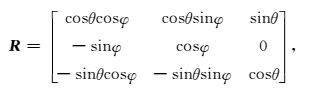(34)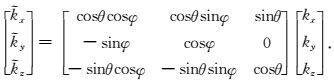(35)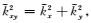可以得到：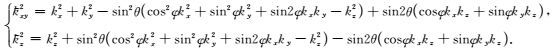(36)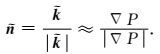(37)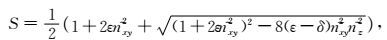(38)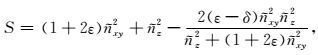(39)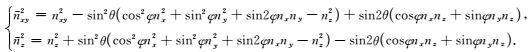(40)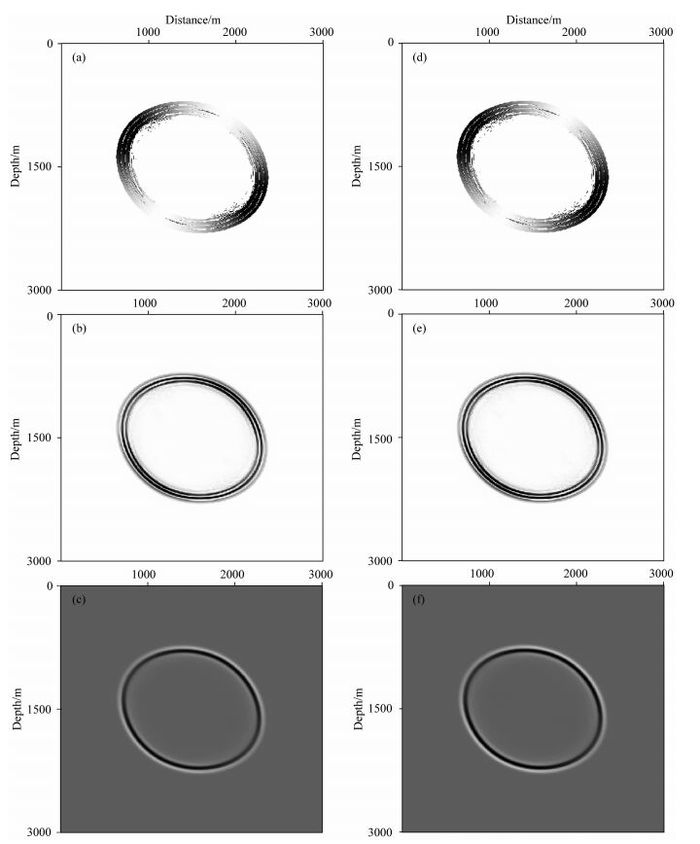图 6 TTI介质中纯qP波正演模拟(方程(36)、(38)、(39)、(40)和(25)) (a) t=0.1 s时沿y轴中心方向标量算子S的二维切片(方程(36)、(38)、(40)和(25))；(b) t=0.1 s时沿y轴中心方向复合算子S∇P的二维切片(方程(36)、(38)、(40)和(25))；(c) t=0.1 s时沿y轴中心方向qP波波场快照的二维切片(方程(36)、(38)、(40)和(25))；(d) t=0.1 s时沿y轴中心方向标量算子S的二维切片(方程(36)、(39)、(40)和(25))；(e) t=0.1 s时沿y轴中心方向复合算子S∇P的二维切片((方程(36)、(39)、(40)和(25))；(f) t=0.1 s时沿y轴中心方向qP波波场快照的二维切片((方程(36)、(39)、(40)和(25)). Fig. 6 Pure qP wave forward modeling in TTI medium (Equations (36), (38), (39), (40) and (25)) (a) 2D seismic slice of scalar operator S along the middle of y direction at time t=0.1 s(Equations (36), (38), (40) and(25)); (b) 2D seismic slice of scalar operator S∇P along the middle of y direction at time t=0.1 s(Equations (36), (38), (40) and (25)); (c) 2D snapshot of qP wavefield along the middle of y direction at time t=0.1 s (Equations (36), (38), (40) and (25)); (d) 2D seismic slice of scalar operator S along the middle of y direction at time t=0.1 s (Equations (36), (39), (40) and (25)); (e) 2D seismic slice of scalar operator S∇P along the middle of y direction at time t=0.1 s (Equations (36), (39), (40) and (25)); (f) 2D snapshot of qP wavefield along the middle of y direction at time t=0.1 s (Equations (36), (39), (40) and (25)).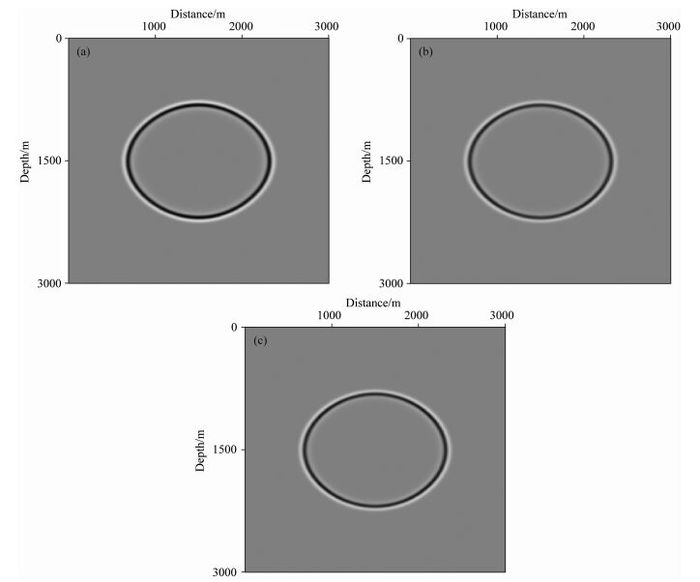图 7 VTI介质中不同纯qP波椭圆分解方程正演模拟(方程(27)、(30)、(31)、(32)和(33)) (a) t=0.1 s时沿y轴中心方向的qP波波场快照二维切片(方程(32)和(33))；(b) t=0.1 s时qP波场沿y轴中心方向的qP波波场快照二维切片(方程(27)和(30))；(c) t=0.1 s时沿y轴中心方向的qP波波场快照二维切片(方程(27)和(31)). Fig. 7 Forward modeling of different pure qP wave elliptical decomposed equations in VTI medium (Equations (27), (30), (31), (32) and (33)) (a) 2D snapshot of qP wavefield along the middle of y direction at time t=0.1 s(Equations (32) and (33)); (b) 2D snapshot of qP wavefield along the middle of y direction at time t=0.1 s(Equations (27) and (30)); (c) 2D snapshot of qP wavefield along the middle of y direction at time t=0.1 s (Equation (27) and (31)).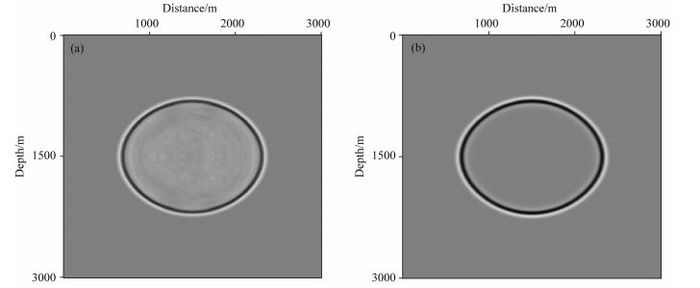图 10 VTI介质中不同纯qP波椭圆分解方程正演模拟(方程(27)、(29)和(30)) (a)未加衰减项(方程(27)和(29))；(b)加衰减项(方程(27)和(30)). Fig. 10 Forward modeling of qP waves using different pure elliptical decomposed equations in VTI medium (Equations (27), (29) and (30)) (a) Equations without attenuating terms added (Equations (27) and (29)); (b) Equations with attenuating terms added (Equations (27) and (30)).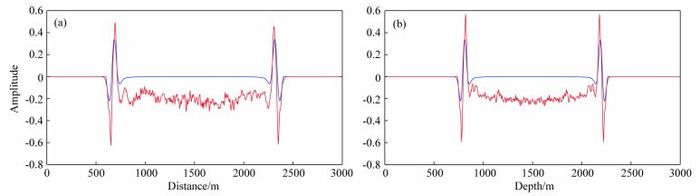图 11 VTI介质中qP波场单道波形分析 (a, b)说明同图 4、5；红线：未加衰减项(方程(27)和(29))；蓝线：加衰减项(方程(27)和(30)). Fig. 11 Waveform analysis of single trace of qP wavefield in VTI medium Red line: equations without attenuating terms (Equations (27) and (29)); Blue line: equations with attenuating terms (Equations (27) and (30)).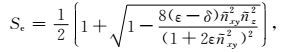(41)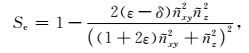(42)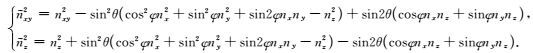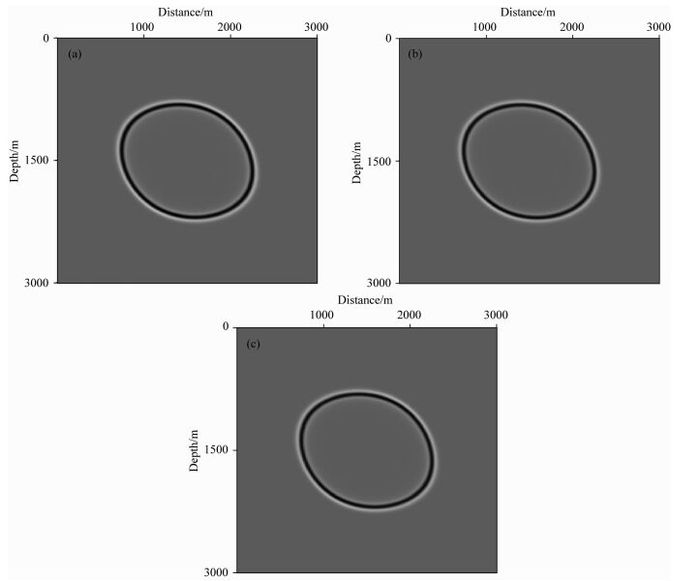图 12 TTI介质中不同纯qP波椭圆分解方程正演模拟(方程(41)、(42)、(40)、(27)、(35)、(36)、(32)和(33)) (a) t=0.1 s时沿y轴中心方向的qP波波场快照二维切片(方程(36)、(32)和(33))；(b) t=0.1 s时沿y轴中心方向的qP波波场快照二维切片(方程(36)、(27)、(41)和(40))；(c) t=0.1 s时沿y轴中心方向的qP波波场快照二维切片(方程(36)、(27)、(42)和(40)). Fig. 12 Forward modeling of different pure qP wave elliptical decomposed equations in TTI medium (Equations (41), (42), (40), (27), (36), (32)和(33)) (a) 2D snapshot of qP wavefield along the middle of y direction at time t=0.1 s(Equations (36), (32) and (33)); (b) 2D snapshot of qP wavefield along the middle of y direction at time t=0.1 s (Equations (36), (27), (41) and (40)); (c) 2D snapshot of qP wavefield along the middle of y direction at time t=0.1 s (Equations (36), (27), (42) and (40)).

4.2 BP模型正演模拟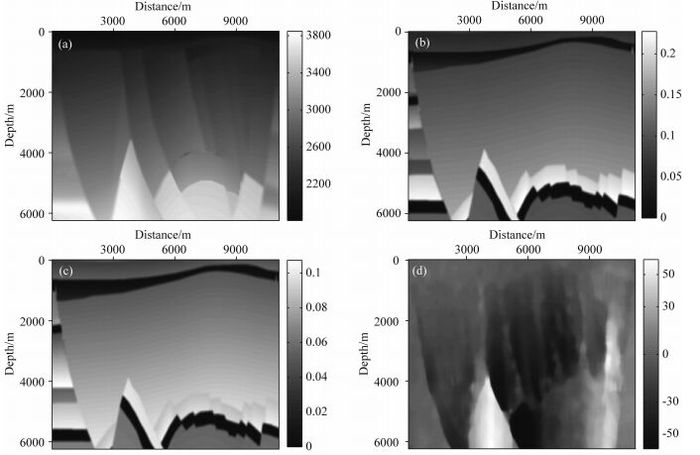图 13 BP部分模型：P波速度场(a)、ε模型(b)、δ模型(c)、θ模型(d) Fig. 13 Part of BP model: P wave velocity(a), ε model(b), δ model(c), θ model(d)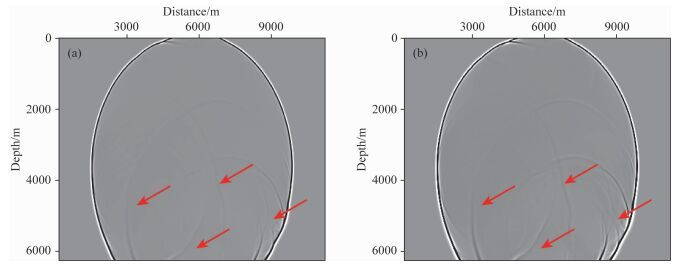图 14 BP模型正演模拟波场快照对比(方程(27)、(40)、(41)和(42)) (a) Xu等(2015)的纯qP波椭圆分解方程；(b)本文的纯qP波椭圆分解方程. Fig. 14 Comparison of snapshots of qP wavefield in BP model(Equations (27), (40), (41) and (42)) (a) Xu′s elliptical decomposed equation; (b) Elliptical decomposed equation proposed in this paper.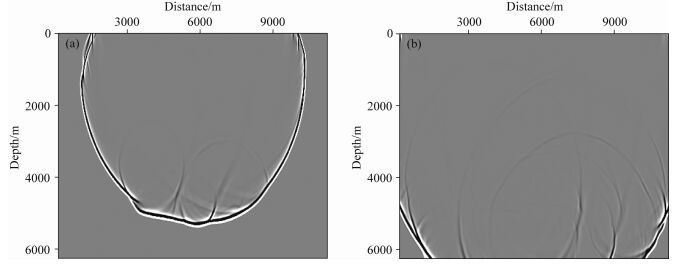图 15 BP模型正演模拟波场快照(方程(27)、(40)和(42)) 震源位置(5500, 0)：(a) t=2.1 s时qP波波场快照；(b) t=2.8 s时qP波波场快照. Fig. 15 Snapshots of qP wavefield in BP model (Equations (27), (40) and (42)) Source at (5500, 0) : (a) Snapshot of qP wavefield at t=2.1 s; (b) Snapshot of qP wavefield at t=2.8 s.
5 逆时偏移中的运用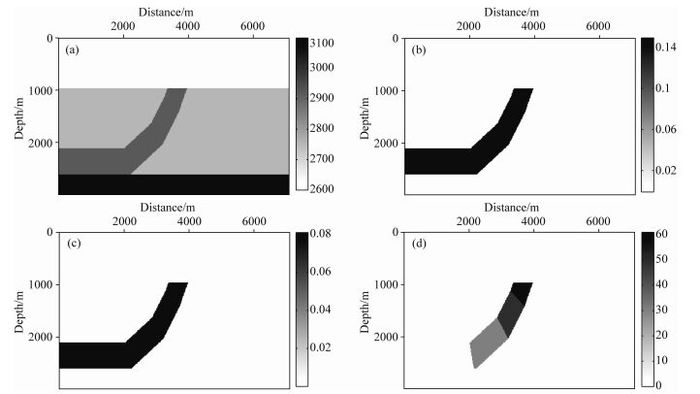图 16 逆冲模型：P波速度场(a)、ε模型(b)、δ模型(c)、θ模型(d) Fig. 16 Thrust model: P wave velocity(a), ε model (b), δ model (c), θ model(d)
5.1 逆冲模型逆时偏移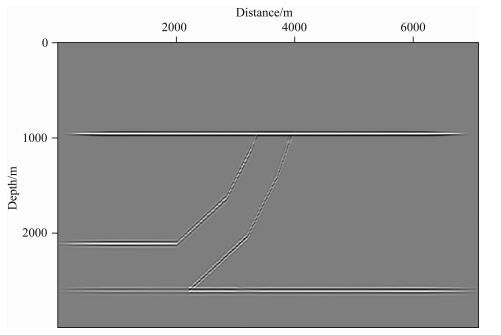图 17 逆冲模型逆时偏移结果 Fig. 17 Reverse time migration results of thrust model

5.2 BP模型逆时偏移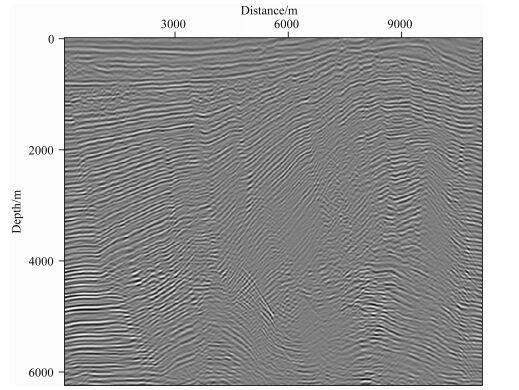图 18 BP模型逆时偏移结果(部分) Fig. 18 Reverse time migration results of part of BP model
6 结论

Xu和Zhou(2014)利用声波近似频散关系，将四阶拟声波方程中的偏微分算子分解成一个Laplace算子和一个标量算子S，构建了二阶纯qP波方程.本文利用Tsvankin(1996)提出的精确频散关系，利用近似展开的方法，同样地将偏微分算子分解，推导出各向异性介质中二阶纯qP波方程.

(1) 这个推导过程无需设置横波速度为0，所以求取的标量算子S更加准确，不用引入衰减项d，就能很好地压制假象干扰，减小数值误差，但是Xu和Zhou(2014)的纯qP波方程人为设置了横波速度为0，只有引入衰减项d，才能很好地压制假象干扰.

(2) 标量算子S的求取需要求解波矢量，波矢量用波场梯度近似，导致了波场振幅的不准确、不均衡，基于Xu等(2015)提出的椭圆分解法，将其应用于本文构建的二阶纯qP波方程中推导出了纯qP波椭圆分解方程，很好地对波场振幅进行了校正，并与Xu等(2015)构建的纯qP波椭圆分解方程对比分析.可以知道，由于本文纯qP波椭圆分解方程中没引入衰减项，校正后的波场振幅值均衡而且更加准确.

(3) 本文将交错网格有限差分算法(参考由Alkan等(2013)提出的DG网格差分算法)和MPML吸收边界条件(Dmitriev and Lisitsa, 2012)应用于均匀TI介质、复杂BP TI模型的正演模拟中，通过qP波波场快照对比和振幅分析，验证了本文方程的正确性、有效性和适用性，并突出了本文构建的qP波椭圆分解方程在振幅校正方面的优势.

(4) 本文将构建的各向异性纯qP波椭圆分解方程应用于叠前逆时偏移技术中，采用归一化互相关成像条件，对TI模型(逆冲模型和BP模型)实现了逆时偏移成像，能够对高陡构造很好地成像，同相轴连续性较好，符合模型真实的构造特征，验证了本文方程在逆时偏移成像中的可行性和对复杂TI介质的适用性.

 Alkan E, Demir V, Elsherbeni A Z, et al. 2013. Double-Grid Finite-Difference Frequency-Domain (DG-FDFD) Method for Scattering from Chiral Objects.: Morgan & Claypool Publishers. Alkhalifah T. 1998. Acoustic approximations for processing in transversely isotropic media. Geophysics, 63(2): 623-631. DOI:10.1190/1.1444361 Alkhalifah T. 2000. An acoustic wave equation for anisotropic media. Geophysics, 65(4): 1239-1250. DOI:10.1190/1.1444815 Cheng J B, Chen M G, Wang T F, et al. 2014. Description of qP-wave propagation in anisotropic media, Part Ⅱ: Separation of pure-mode scalar waves. Chinese Journal of Geophysics, 57(10): 3389-3401. DOI:10.6038/cjg20141025 Cheng J B, Kang W, Wang T F. 2013. Description of qP-wave propagation in anisotropic media, Part Ⅰ: Pseudo-pure-mode wave equations. Chinese Journal of Geophysics, 56(10): 3474-3486. DOI:10.6038/cjg20131022 Dellinger J, Etgen J. 1990. Wave-field separation in two-dimension anisotropic media. Geophysics, 55(7): 914-919. DOI:10.1190/1.1442906 Dmitriev M N, Lisitsa V V. 2012. Application of M-PML absorbing boundary conditions to the numerical simulation of wave propagation in anisotropic media. Part Ⅱ: Stability. Numer. Analys. Appl., 5(1): 36-44. DOI:10.1134/S1995423912010041 Du X, Bancroft J C, Lines L R. 2005. Reverse-time migration for tilted TI media.//2005 SEG Annual Meeting. Houston, Texas: SEG, 1930-1933. Duveneck E, Milcik P, Bakker P M, et al. 2008. Acoustic VTI wave equations and their application for anisotropic reverse-time migration.//2008 SEG Annual Meeting. Las Vegas, Nevada: SEG, 2186-2190. Fletcher R P, Du X, Fowler P J. 2009. Reverse time migration in tilted transversely isotropic (TTI) media. Geophysics, 74(6): WCA179-WCA187. DOI:10.1190/1.3269902 Fowler P J, Du X, Fletcher R P. 2010. Coupled equations for reverse time migration in transversely isotropic media. Geophysics, 75(1): S11-S22. DOI:10.1190/1.3294572 Ge Z, Pestana R C, Stoffa P L. 2011. An acoustic wave equation for pure P wave in 2D TTI media.//81st Annual International Meeting. SEG, 168-173. Grechka V, Zhang L B, Rector J W. 2004. Shear waves in acoustic anisotropic media. Geophysics, 69(2): 576-582. DOI:10.1190/1.1707077 Hestholm S. 2009. Acoustic VTI modeling using high-order finite differences. Geophysics, 74(5): T67-T73. DOI:10.1190/1.3157242 Liu F Q, Morton S A, Jiang S S, et al. 2009. Decoupled wave equations for P and SV waves in an acoustic VTI media.//2009 SEG Annual Meeting. Houston, Texas: SEG, 2844-2848. Thomsen L. 1986. Weak elastic anisotropy. Geophysics, 51(10): 1954-1966. DOI:10.1190/1.1442051 Tsvankin I. 1996. P-wave signatures and notation for transversely isotropic media: an overview. Geophysics, 61(2): 467-483. DOI:10.1190/1.1443974 Wu G C, Liang K. 2005. Quasi P-wave forward modeling in frequency-space domain in VTI media. Oil Geophysical Prospecting, 40(5): 535-545. Wu G C, Liang K. 2007. High precision finite difference operators for qP wave equation in VTI media. Progress in Geophysics, 22(3): 896-904. Wu G C, Liang K, Yin X Y. 2010. The analysis of phase velocity and polarization feature for elastic wave in TTI media. Chinese Journal of Geophysics, 53(8): 1914-1923. Xu S, Zhou H B. 2014. Accurate simulations of pure quasi-P-waves in complex anisotropic media. Geophysics, 79(6): T341-T348. DOI:10.1190/geo2014-0242.1 Xu S, Tang B, Mu J, et al. 2015. Quasi-P wave propagation with an elliptic differential operator.//2015 SEG Annual Meeting. New Orleans, Louisiana: SEG, 4380-4384. Yan J, Sava P. 2009. Elastic wave-mode separation for VTI media. Geophysics, 74(5): WB19-WB32. DOI:10.1190/1.3184014 Zhan G, Pestana R C, Stoffa P L. 2012. Decoupled equations for reverse time migration in tilted transversely isotropic media. Geophysics, 77(2): T37-T45. DOI:10.1190/geo2011-0175.1 Zhang H Z, Zhang G Q, Zhang Y. 2009. Removing S-wave noise in TTI reverse time migration.//79th Annual International Meeting, SEG Expanded Abstracts. SEG, 2849-2853. Zhang Y, Wu G C. 2013. Methods of removing pseudo SV-wave artifacts in TTI media qP-wave reverse-time migration. Chinese Journal of Geophysics, 56(6): 2065-2076. DOI:10.6038/cjg20130627 Zhang Y, Zhang Houzhu, Zhang Guanquan. 2011. A stable TTI reverse time migration and its implementation. Geophysics, 76(3): WA3-WA11. DOI:10.1190/1.3554411 Zhou H B, Zhang G, Bloor R. 2006a. An anisotropic acoustic wave equation for VTI media.//68th Annual International Conference and Exhibition. SPE, EAGE. Zhou H B, Zhang G Q, Bloor R. 2006b. An anisotropic acoustic wave equation for modeling and migration in 2D TTI media.//2006 SEG Annual Meeting. New Orleans, Louisiana: SEG, 194-198. 程玖兵, 康玮, 王腾飞. 2013. 各向异性介质qP波传播描述I:伪纯模式波动方程. 地球物理学报, 56(10): 3474–3486. DOI:10.6038/cjg20131022 程玖兵, 陈茂根, 王腾飞, 等. 2014. 各向异性介质qP波传播描述Ⅱ:分离纯模式标量波. 地球物理学报, 57(10): 3389–3401. DOI:10.6038/cjg20141025 吴国忱, 梁锴. 2005. VTI介质频率-空间域准P波正演模拟. 石油地球物理勘探, 40(5): 535–545. 吴国忱, 梁锴. 2007. VTI介质qP波方程高精度有限差分算子. 地球物理学进展, 22(3): 896–904. 吴国忱, 梁锴, 印兴耀. 2010. TTI介质弹性波相速度与偏振特征分析. 地球物理学报, 53(8): 1914–1923. 张岩, 吴国忱. 2013. TTI介质qP波逆时偏移中伪横波噪声压制方法. 地球物理学报, 56(6): 2065–2076. DOI:10.6038/cjg20130627